In 2018, Kaggle surveyed 23,859 data scientists to learn more about the current state of data science. The data they collected and more information about the survey can be found here. In this article, I dive into the dataset to see what we can learn about the current state of data scientists in the world.

My findings paint a clear picture as to why Jack and I designed Datafied the way we did. I hope you enjoy the read!

Below, I first import necessary libraries and the data.

# Python Libraries
import pandas as pd
import numpy as np
import seaborn as sns
import matplotlib.pyplot as plt



The first thing that caught my attention is the disproportionate distribution of genders in the field of data science.

sns.countplot(x='Q1', data=mc_18[(mc_18.Q1 == "Female") | (mc_18.Q1 == "Male")])
plt.title("Distribution of Genders 2018", fontsize=14)
plt.xlabel("Gender", fontsize=14)
plt.show()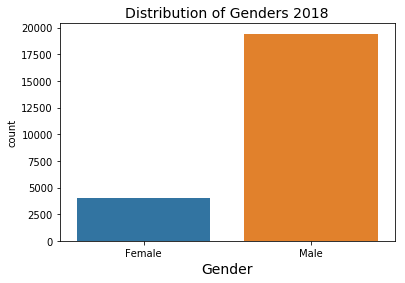I've read articles before that talked about the gender gap in tech, but I didn't know it looked like this. Therefore, our first goal with designing Datafied was to make a platform that can appeal everyone. It's extremely important to us that every single person, no matter your gender, can benefit from the value that data science provides to the world.

The next thing I look into is the age of data scientists. Below is a countplot of the results.

plt.figure(figsize=(10,5))
sns.countplot(x='Q2', data=mc_18.sort_values('Q2'))
plt.title("Age of Data Scientists", fontsize=14)
plt.xlabel("Age Group", fontsize=14)
plt.ylabel("Count", fontsize=14)
plt.show()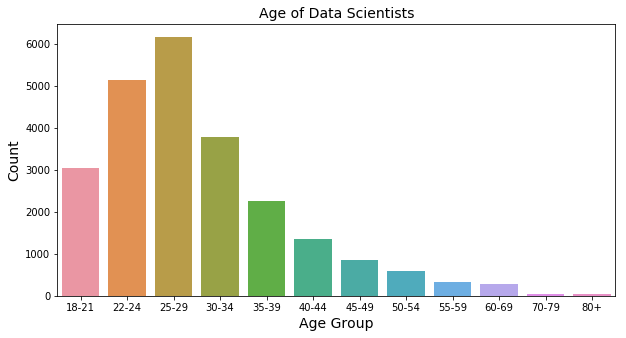It looks like on average, data scientists tend to be in their mid to late 20s. It also appears that few 50+ year olds are interested in data science. If we are going to build a platform that appeals to everyone, it can't just be used by 20 year olds. Therefore, our next goal with Datafied is to focus on communication, not technicality. We'd like to make the above plot look more uniform. If we as data scientists can clearly explain our work in a way that can appeal to someone who doesn't understand the tech world as well, then we can spread the benefit of data science to a much larger audience.

Next, I look at the different countries of data scientists who filled out the survey.

mc_18.Q3.value_counts().plot(kind='bar', figsize=(14,7))
plt.title("Number of Data Scientists Per Country", fontsize=14)
plt.xlabel("Country", fontsize=14)
plt.ylabel("Count", fontsize=14)
plt.show()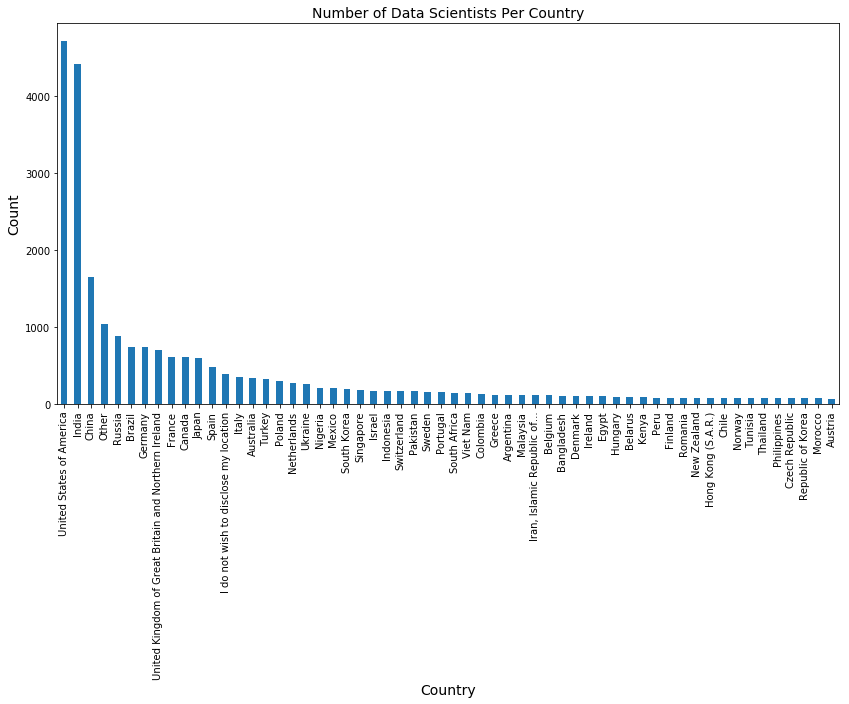United States has the largest number of data scientist, but not by far. India is a close second and there are a ton of other countries beginning to produce data scientists. Seeing this made us realize that if we truly want to build a platform for everyone, we can't limit ourselves to one country. That is why we use the url extension ".world". Datafied is a platform for the entire world to benefit from data science.

I'm also curious to look at the most popular programming languages used by data scientists.

sns.countplot(mc_18.Q17, order = mc_18.Q17.value_counts().index)
plt.title("Programming languages used most often", fontsize=14)
plt.xticks(rotation=90)
plt.xlabel("Language", fontsize=14)
plt.ylabel("Count", fontsize=14)
plt.show()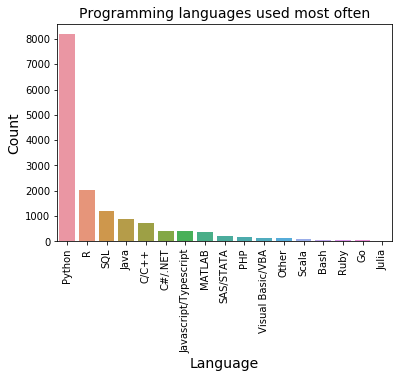Looking at the above countplot, Python is by far the programming language of choice in the data science community. Therefore, we instantly knew that we needed to support softwares that used python.

Next, I looked at the most popular data science softwares.

data = {'software':['Jupyter', 'R Studio', 'PyCharm', 'MATLAB', 'Visual Code Studio'],
'proportion': [np.count_nonzero(mc_18.Q13_Part_1.fillna(0))/len(mc_18.Q13_Part_1),
np.count_nonzero(mc_18.Q13_Part_2.fillna(0))/len(mc_18.Q13_Part_2),
np.count_nonzero(mc_18.Q13_Part_3.fillna(0))/len(mc_18.Q13_Part_3),
np.count_nonzero(mc_18.Q13_Part_7.fillna(0))/len(mc_18.Q13_Part_7),
np.count_nonzero(mc_18.Q13_Part_4.fillna(0))/len(mc_18.Q13_Part_4)]}
plt.figure(figsize=(8,5))
sns.barplot(x='software', y='proportion', data=pd.DataFrame(data))
plt.title("Proportion of data scientists who have used X software in the last 5 years")
plt.xlabel("Software", fontsize=14)
plt.ylabel("Percent (%)", fontsize=14)
plt.show()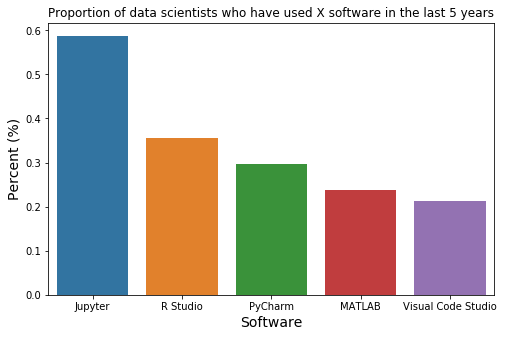Looking at the above barplot, Jupyter is the most popular software used in data science. We wanted to make sure that our website tailors to the most popular software in the world and also uses Python, the most common data science programming language in the world, and that is why we chose to support Jupyter. It was an obvious choice for us.

The last thing that I looked were the most common methods that data scientists used to learn data science.

data = {'learning_type':['self taught', 'online courses', 'university', 'Work', 'Kaggle competitions', 'other'],
'proportion': [np.mean(mc_18.Q35_Part_1)/100,
np.mean(mc_18.Q35_Part_2)/100,
np.mean(mc_18.Q35_Part_4)/100,
np.mean(mc_18.Q35_Part_3)/100,
np.mean(mc_18.Q35_Part_5)/100,
np.mean(mc_18.Q35_Part_6)/100]}
plt.figure(figsize=(8,5))
sns.barplot(x='learning_type', y='proportion', data=pd.DataFrame(data))
plt.title("Percentage of current ML/Data Science Training")
plt.xlabel("Type")
plt.ylabel("Percent (%)")
plt.show()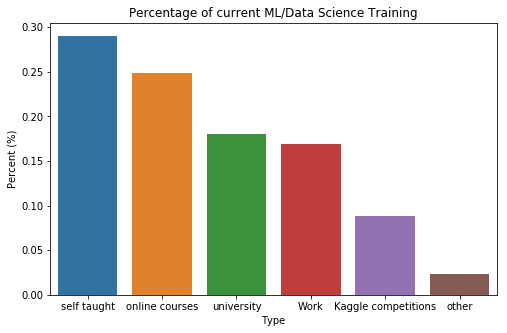I learned that most data scientists learn through "self taught" methods. Because of this, we wanted to build a platform that can help data scientists learn on their own.

In summary, Jack and I were seeking to build a data science product for everyone -- no matter your gender, age, or country of origin -- that supports the most popular tools used in data science -- Python and Jupyter -- that helps data scientists learn in a way they are already familiar with -- self-taught. Our result: Datafied.world.

We hope you enjoy our website and are open to feedback to help turn it into a site that you love. Don't hesitate to email me (colestriler at gmail dot com) for any feedback or questions you have about the site!